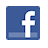Games
Problems
Go Pro!

# Triangular Operation

Pro Problems > Math > Number and Quantity > Number Theory > Star Operations

## Triangular Operation

The arrangement
a
b
c
means a - (b x c). For example,
20
3
4
means 20 - (3 x 4) = 20 - 12 = 8.

Find the value of
x
y
z
y
x
z
z
x
y
, if x = 10, y = 7, and z = 2.

Presentation mode
Problem by Mr. Twitchell

## Solution

In order to make it feasible for teachers to use these problems in their classwork, no solutions are publicly visible, so students cannot simply look up the answers. If you would like to view the solutions to these problems, you must have a Virtual Classroom subscription.Assign this problem
Click here to assign this problem to your students.

## Similar Problems

### Commutative Operation

The operation * is defined as follows: x * y = (ax + b)(by + a), for some real numbers a and b. Under what conditions is this operation commutative?

### Diamond Operation

If the operation ♦ is defined as follows: a ♦ b = a2 - b2 , and18 ♦ (x ♦ 8) = 6 ♦ 1, find all possible values of x.

### Hashtag Operation

The "hashtag operation" is defined as follows: a # b =
a + b
a
+
a + b
b
.

If x # 7 =
16
3
, find all possible values of x.

### Guess the Star

There exists a star (*) operation for which the following equations are true:

2 * 3 = 10
5 * 2 = 35
3 * 6 = 27
8 * 7 = 120

Find a star operation rule which satisfies all of these equations, and then use your rule to calculate the following:

10 * 11 = ?

### h and k

x * y = x2 - y2 + kx - hy. If 5 * 2 = 48 and 4 * 3 = 30, find the ordered pair (h, k).

### Star Operation with Cubes

If the operation (*) is defined as follows: a * b =
a3 + b3
a2 - ab + b2
, find the value of (7 * 3) * 4.

### Fractional Operation

The operation a » b is defined as follows: a » b =
a
a + 1
+
b
b - 3
. Find the value of a such that a »  a =
12
5
.

### Non-Commutative Operation

If the operation a ♦ b is defined as follows: a ♦ b =
a
b
+ a, find the value of x such that:

x  ♦ 2x = 2x ♦ x.

Note that this operation is not commutative; the order of operands cannot be exchanged without altering the result of the operation.

### Star and Pound Operations

If a * b = (a + b)2, and a # b = (a - b)2, find x such that (2 * x) # (x * 1) = 81

# Featured Games on This Site

Match color, font, and letter in this strategy game
Trap all the dots in this problem-solving puzzle

# Blogs on This SiteReviews and book lists - books we love!The site administrator fields questions from visitors.Like us on Facebook to get updates about new resources